# Calculus Symbols

Symbol
Symbol Name
Meaning / definition
Example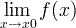limit
limit value of a function
ε
epsilon
represents a very small number, near zero
ε →0
e
e constant / Euler’s number
e = 2.718281828…
e = lim (1+1/x)x ,x→∞
y
derivative
derivative – Leibniz’s notation
(3x3)’ = 9x2
y
second derivative
derivative of derivative
(3x3)” = 18x
y(n)
nth derivative
n times derivation
(3x3)(3) = 18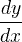derivative
derivative – Lagrange’s notation
d(3x3)/dx = 9x2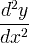second derivative
derivative of derivative
d2(3x3)/dx2 = 18x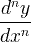nth derivative
n times derivationtime derivative
derivative by time – Newton notation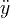time second derivative
derivative of derivative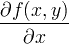partial derivative
∂(x2+y2)/∂x = 2x
integral
opposite to derivation
double integral
integration of function of 2 variables
triple integral
integration of function of 3 variables
closed contour / line integral
closed surface integral
closed volume integral
[a,b]
closed interval
[a,b] = {x | a x b}
(a,b)
open interval
(a,b) = {x | a < x < b}
i
imaginary unit
i ≡ √-1
z = 3 + 2i
z*
complex conjugate
z = a+biz*=abi
z* = 3 + 2i
z
complex conjugate
z = a+biz = abi
z = 3 + 2i
nabla / del
f (x,y,z)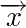vectorunit vector
x * y
convolution
y(t) = x(t) * h(t)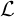Laplace transform
F(s) ={f (t)}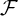Fourier transform
X(ω) ={f (t)}
δ
delta function
lemniscate
infinity symbol